Chemistry

# 13.4Equilibrium Calculations

Chemistry13.4 Equilibrium Calculations

### Learning Objectives

By the end of this section, you will be able to:
• Write equations representing changes in concentration and pressure for chemical species in equilibrium systems
• Use algebra to perform various types of equilibrium calculations

We know that at equilibrium, the value of the reaction quotient of any reaction is equal to its equilibrium constant. Thus, we can use the mathematical expression for Q to determine a number of quantities associated with a reaction at equilibrium or approaching equilibrium. While we have learned to identify in which direction a reaction will shift to reach equilibrium, we want to extend that understanding to quantitative calculations. We do so by evaluating the ways that the concentrations of products and reactants change as a reaction approaches equilibrium, keeping in mind the stoichiometric ratios of the reaction. This algebraic approach to equilibrium calculations will be explored in this section.

Changes in concentrations or pressures of reactants and products occur as a reaction system approaches equilibrium. In this section we will see that we can relate these changes to each other using the coefficients in the balanced chemical equation describing the system. We use the decomposition of ammonia as an example.

On heating, ammonia reversibly decomposes into nitrogen and hydrogen according to this equation:

$2NH3(g)⇌N2(g)+3H2(g)2NH3(g)⇌N2(g)+3H2(g)$
13.65

If a sample of ammonia decomposes in a closed system and the concentration of N2 increases by 0.11 M, the change in the N2 concentration, Δ[N2], the final concentration minus the initial concentration, is 0.11 M. The change is positive because the concentration of N2 increases.

The change in the H2 concentration, Δ[H2], is also positive—the concentration of H2 increases as ammonia decomposes. The chemical equation tells us that the change in the concentration of H2 is three times the change in the concentration of N2 because for each mole of N2 produced, 3 moles of H2 are produced.

$Δ[H2]=3×Δ[N2]Δ[H2]=3×Δ[N2]$
13.66
$=3×(0.11M)=0.33M=3×(0.11M)=0.33M$
13.67

The change in concentration of NH3, Δ[NH3], is twice that of Δ[N2]; the equation indicates that 2 moles of NH3 must decompose for each mole of N2 formed. However, the change in the NH3 concentration is negative because the concentration of ammonia decreases as it decomposes.

$Δ[NH3]=−2×Δ[N2]=−2×(0.11M)=−0.22MΔ[NH3]=−2×Δ[N2]=−2×(0.11M)=−0.22M$
13.68

We can relate these relationships directly to the coefficients in the equation

$2NH3(g)⇌N2(g)+3H2(g)Δ[NH3]=−2×Δ[N2]Δ[N2]=0.11MΔ[H2]=3×Δ[N2]2NH3(g)⇌N2(g)+3H2(g)Δ[NH3]=−2×Δ[N2]Δ[N2]=0.11MΔ[H2]=3×Δ[N2]$
13.69

Note that all the changes on one side of the arrows are of the same sign and that all the changes on the other side of the arrows are of the opposite sign.

If we did not know the magnitude of the change in the concentration of N2, we could represent it by the symbol x.

$Δ[N2]=xΔ[N2]=x$
13.70

The changes in the other concentrations would then be represented as:

$Δ[H2]=3×Δ[N2]=3xΔ[H2]=3×Δ[N2]=3x$
13.71
$Δ[NH3]=−2×Δ[N2]=−2xΔ[NH3]=−2×Δ[N2]=−2x$
13.72

The coefficients in the Δ terms are identical to those in the balanced equation for the reaction.

$2NH3(g)⇌N2(g)+3H2(g)−2xx3x2NH3(g)⇌N2(g)+3H2(g)−2xx3x$
13.73

The simplest way for us to find the coefficients for the concentration changes in any reaction is to use the coefficients in the balanced chemical equation. The sign of the coefficient is positive when the concentration increases; it is negative when the concentration decreases.

### Example 13.5

#### Determining Relative Changes in Concentration

Complete the changes in concentrations for each of the following reactions.

(a) $C2H2(g)+2Br2(g)⇌C2H2Br4(g)x__________C2H2(g)+2Br2(g)⇌C2H2Br4(g)x__________$

(b) $I2(aq)+I−(aq)⇌I3−(aq)__________xI2(aq)+I−(aq)⇌I3−(aq)__________x$

(c) $C3H8(g)+5O2(g)⇌3CO2(g)+4H2O(g)x_______________C3H8(g)+5O2(g)⇌3CO2(g)+4H2O(g)x_______________$

#### Solution

(a) $C2H2(g)+2Br2(g)⇌C2H2Br4(g)x2x−xC2H2(g)+2Br2(g)⇌C2H2Br4(g)x2x−x$

(b) $I2(aq)+I−(aq)⇌I3−(aq)−x−xxI2(aq)+I−(aq)⇌I3−(aq)−x−xx$

(c) $C3H8(g)+5O2(g)⇌3CO2(g)+4H2O(g)x5x−3x−4xC3H8(g)+5O2(g)⇌3CO2(g)+4H2O(g)x5x−3x−4x$

Complete the changes in concentrations for each of the following reactions:

(a) $2SO2(g)+O2(g)⇌2SO3(g)_____x_____2SO2(g)+O2(g)⇌2SO3(g)_____x_____$

(b) $C4H8(g)⇌2C2H4(g)_____−2xC4H8(g)⇌2C2H4(g)_____−2x$

(c) $4NH3(g)+7H2O(g)⇌4NO2(g)+6H2O(g)____________________4NH3(g)+7H2O(g)⇌4NO2(g)+6H2O(g)____________________$

(a) 2x, x, −2x; (b) x, −2x; (c) 4x, 7x, −4x, −6x or −4x, −7x, 4x, 6x

### Calculations Involving Equilibrium Concentrations

Because the value of the reaction quotient of any reaction at equilibrium is equal to its equilibrium constant, we can use the mathematical expression for Qc (i.e., the law of mass action) to determine a number of quantities associated with a reaction at equilibrium. It may help if we keep in mind that Qc = Kc (at equilibrium) in all of these situations and that there are only three basic types of calculations:

1. Calculation of an equilibrium constant. If concentrations of reactants and products at equilibrium are known, the value of the equilibrium constant for the reaction can be calculated.
2. Calculation of missing equilibrium concentrations. If the value of the equilibrium constant and all of the equilibrium concentrations, except one, are known, the remaining concentration can be calculated.
3. Calculation of equilibrium concentrations from initial concentrations. If the value of the equilibrium constant and a set of concentrations of reactants and products that are not at equilibrium are known, the concentrations at equilibrium can be calculated.

A similar list could be generated using QP, KP, and partial pressure. We will look at solving each of these cases in sequence.

#### Calculation of an Equilibrium Constant

In order to calculate an equilibrium constant, enough information must be available to determine the equilibrium concentrations of all reactants and products. Armed with the concentrations, we can solve the equation for Kc, as it will be the only unknown.

Example 13.2 showed us how to determine the equilibrium constant of a reaction if we know the concentrations of reactants and products at equilibrium. The following example shows how to use the stoichiometry of the reaction and a combination of initial concentrations and equilibrium concentrations to determine an equilibrium constant. This technique, commonly called an ICE chart—for Initial, Change, and Equilibrium–will be helpful in solving many equilibrium problems. A chart is generated beginning with the equilibrium reaction in question. The initial concentrations of the reactants and products are provided in the first row of the ICE table (these essentially time-zero concentrations that assume no reaction has taken place). The next row of the table contains the changes in concentrations that result when the reaction proceeds toward equilibrium (don’t forget to account for the reaction stoichiometry). The last row contains the concentrations once equilibrium has been reached.

### Example 13.6

#### Calculation of an Equilibrium Constant

Iodine molecules react reversibly with iodide ions to produce triiodide ions.
$I2(aq)+I−(aq)⇌I3−(aq)I2(aq)+I−(aq)⇌I3−(aq)$
13.74

If a solution with the concentrations of I2 and I both equal to 1.000 $××$ 10−3 M before reaction gives an equilibrium concentration of I2 of 6.61 $××$ 10−4 M, what is the equilibrium constant for the reaction?

#### Solution

We will begin this problem by calculating the changes in concentration as the system goes to equilibrium. Then we determine the equilibrium concentrations and, finally, the equilibrium constant. First, we set up a table with the initial concentrations, the changes in concentrations, and the equilibrium concentrations using −x as the change in concentration of I2.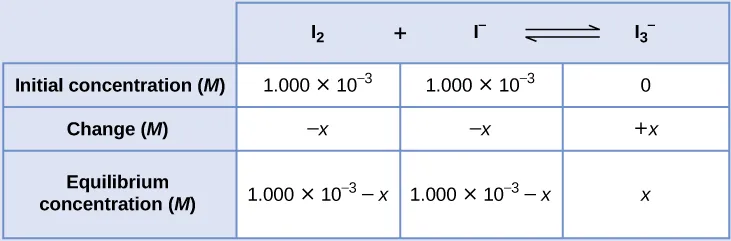Since the equilibrium concentration of I2 is given, we can solve for x. At equilibrium the concentration of I2 is 6.61 $××$ 10−4 M so that

$1.000×10−3−x=6.61×10−41.000×10−3−x=6.61×10−4$
13.75
$x=1.000×10−3−6.61×10−4x=1.000×10−3−6.61×10−4$
13.76
$=3.39×10−4M=3.39×10−4M$
13.77

Now we can fill in the table with the concentrations at equilibrium.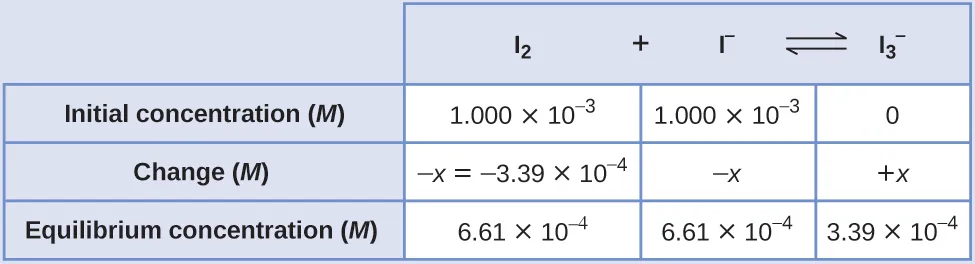We now calculate the value of the equilibrium constant.

$Kc=Qc=[I3−][I2][I−]Kc=Qc=[I3−][I2][I−]$
13.78
$=3.39×10−4M(6.61×10−4M)(6.61×10−4M)=776=3.39×10−4M(6.61×10−4M)(6.61×10−4M)=776$
13.79

Ethanol and acetic acid react and form water and ethyl acetate, the solvent responsible for the odor of some nail polish removers.
$C2H5OH+CH3CO2H⇌CH3CO2C2H5+H2OC2H5OH+CH3CO2H⇌CH3CO2C2H5+H2O$
13.80

When 1 mol each of C2H5OH and CH3CO2H are allowed to react in 1 L of the solvent dioxane, equilibrium is established when $1313$ mol of each of the reactants remains. Calculate the equilibrium constant for the reaction. (Note: Water is not a solvent in this reaction.)

Kc = 4

#### Calculation of a Missing Equilibrium Concentration

If we know the equilibrium constant for a reaction and know the concentrations at equilibrium of all reactants and products except one, we can calculate the missing concentration.

### Example 13.7

#### Calculation of a Missing Equilibrium Concentration

Nitrogen oxides are air pollutants produced by the reaction of nitrogen and oxygen at high temperatures. At 2000 °C, the value of the equilibrium constant for the reaction, $N2(g)+O2(g)⇌2NO(g),N2(g)+O2(g)⇌2NO(g),$ is 4.1 $××$ 10−4. Calculate the equilibrium concentration of NO(g) in air at 1 atm pressure and 2000 °C. The equilibrium concentrations of N2 and O2 at this pressure and temperature are 0.036 M and 0.0089 M, respectively.

#### Solution

We are given all of the equilibrium concentrations except that of NO. Thus, we can solve for the missing equilibrium concentration by rearranging the equation for the equilibrium constant.
$Kc=Qc=[NO]2[N2][O2]Kc=Qc=[NO]2[N2][O2]$
13.81
$[NO]2=Kc[N2][O2][NO]2=Kc[N2][O2]$
13.82
$[NO]=Kc[N2][O2][NO]=Kc[N2][O2]$
13.83
$=(4.1×10−4)(0.036)(0.0089)=(4.1×10−4)(0.036)(0.0089)$
13.84
$=1.31×10−7=1.31×10−7$
13.85
$=3.6×10−4=3.6×10−4$
13.86

Thus [NO] is 3.6 $××$ 10−4 mol/L at equilibrium under these conditions.

We can check our answer by substituting all equilibrium concentrations into the expression for the reaction quotient to see whether it is equal to the equilibrium constant.

$Qc=[NO]2[N2][O2]Qc=[NO]2[N2][O2]$
13.87
$=(3.6×10−4)2(0.036)(0.0089)=(3.6×10−4)2(0.036)(0.0089)$
13.88
$Qc=4.0×10−4=KcQc=4.0×10−4=Kc$
13.89

The answer checks; our calculated value gives the equilibrium constant within the error associated with the significant figures in the problem.

The equilibrium constant for the reaction of nitrogen and hydrogen to produce ammonia at a certain temperature is 6.00 $××$ 10−2. Calculate the equilibrium concentration of ammonia if the equilibrium concentrations of nitrogen and hydrogen are 4.26 M and 2.09 M, respectively.

1.53 mol/L

#### Calculation of Equilibrium Concentrations from Initial Concentrations

If we know the equilibrium constant for a reaction and a set of concentrations of reactants and products that are not at equilibrium, we can calculate the changes in concentrations as the system comes to equilibrium, as well as the new concentrations at equilibrium. The typical procedure can be summarized in four steps.

1. Determine the direction the reaction proceeds to come to equilibrium.
1. Write a balanced chemical equation for the reaction.
2. If the direction in which the reaction must proceed to reach equilibrium is not obvious, calculate Qc from the initial concentrations and compare to Kc to determine the direction of change.
2. Determine the relative changes needed to reach equilibrium, then write the equilibrium concentrations in terms of these changes.
1. Define the changes in the initial concentrations that are needed for the reaction to reach equilibrium. Generally, we represent the smallest change with the symbol x and express the other changes in terms of the smallest change.
2. Define missing equilibrium concentrations in terms of the initial concentrations and the changes in concentration determined in (a).
3. Solve for the change and the equilibrium concentrations.
1. Substitute the equilibrium concentrations into the expression for the equilibrium constant, solve for x, and check any assumptions used to find x.
2. Calculate the equilibrium concentrations.
4. Check the arithmetic.
1. Check the calculated equilibrium concentrations by substituting them into the equilibrium expression and determining whether they give the equilibrium constant.
Sometimes a particular step may differ from problem to problem—it may be more complex in some problems and less complex in others. However, every calculation of equilibrium concentrations from a set of initial concentrations will involve these steps.
In solving equilibrium problems that involve changes in concentration, sometimes it is convenient to set up an ICE table, as described in the previous section.

### Example 13.8

#### Calculation of Concentration Changes as a Reaction Goes to Equilibrium

Under certain conditions, the equilibrium constant for the decomposition of PCl5(g) into PCl3(g) and Cl2(g) is 0.0211. What are the equilibrium concentrations of PCl5, PCl3, and Cl2 if the initial concentration of PCl5 was 1.00 M?

#### Solution

Use the stepwise process described earlier.
1. Step 1.

Determine the direction the reaction proceeds.

The balanced equation for the decomposition of PCl5 is

$PCl5(g)⇌PCl3(g)+Cl2(g)PCl5(g)⇌PCl3(g)+Cl2(g)$
13.90

Because we have no products initially, Qc = 0 and the reaction will proceed to the right.

2. Step 2.

Determine the relative changes needed to reach equilibrium, then write the equilibrium concentrations in terms of these changes.

Let us represent the increase in concentration of PCl3 by the symbol x. The other changes may be written in terms of x by considering the coefficients in the chemical equation.

$PCl5(g)⇌PCl3(g)+Cl2(g)−xxxPCl5(g)⇌PCl3(g)+Cl2(g)−xxx$
13.91

The changes in concentration and the expressions for the equilibrium concentrations are: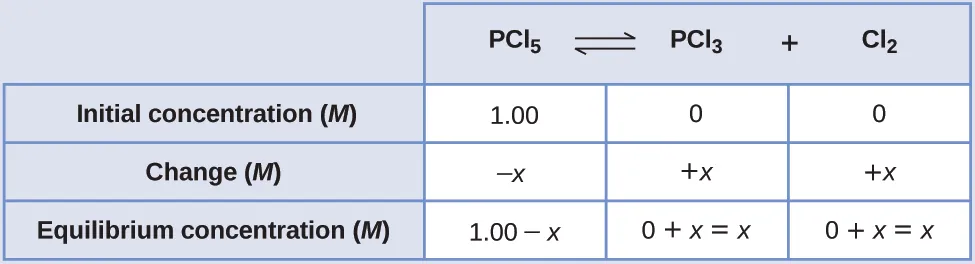3. Step 3.

Solve for the change and the equilibrium concentrations.

Substituting the equilibrium concentrations into the equilibrium constant equation gives

$Kc=[PCl3][Cl2][PCl5]=0.0211Kc=[PCl3][Cl2][PCl5]=0.0211$
13.92
$=(x)(x)(1.00−x)=(x)(x)(1.00−x)$
13.93

This equation contains only one variable, x, the change in concentration. We can write the equation as a quadratic equation and solve for x using the quadratic formula.

$0.0211=(x)(x)(1.00−x)0.0211=(x)(x)(1.00−x)$
13.94
$0.0211(1.00−x)=x20.0211(1.00−x)=x2$
13.95
$x2+0.0211x−0.0211=0x2+0.0211x−0.0211=0$
13.96

Appendix B shows us an equation of the form ax2 + bx + c = 0 can be rearranged to solve for x:

$x=−b±b2−4ac2ax=−b±b2−4ac2a$
13.97

In this case, a = 1, b = 0.0211, and c = −0.0211. Substituting the appropriate values for a, b, and c yields:

$x=−0.0211±(0.0211)2−4(1)(−0.0211)2(1)x=−0.0211±(0.0211)2−4(1)(−0.0211)2(1)$
13.98
$=−0.0211±(4.45×10−4)+(8.44×10−2)2=−0.0211±(4.45×10−4)+(8.44×10−2)2$
13.99
$=−0.0211±0.2912=−0.0211±0.2912$
13.100

Hence

$x=−0.0211+0.2912=0.135x=−0.0211+0.2912=0.135$
13.101

or

$x=−0.0211−0.2912=−0.156x=−0.0211−0.2912=−0.156$
13.102

Quadratic equations often have two different solutions, one that is physically possible and one that is physically impossible (an extraneous root). In this case, the second solution (−0.156) is physically impossible because we know the change must be a positive number (otherwise we would end up with negative values for concentrations of the products). Thus, x = 0.135 M.

The equilibrium concentrations are

$[PCl5]=1.00−0.135=0.87M[PCl5]=1.00−0.135=0.87M$
13.103
$[PCl3]=x=0.135M[PCl3]=x=0.135M$
13.104
$[Cl2]=x=0.135M[Cl2]=x=0.135M$
13.105
4. Step 4.

Check the arithmetic.

Substitution into the expression for Kc (to check the calculation) gives

$Kc=[PCl3][Cl2][PCl5]=(0.135)(0.135)0.87=0.021Kc=[PCl3][Cl2][PCl5]=(0.135)(0.135)0.87=0.021$
13.106

The equilibrium constant calculated from the equilibrium concentrations is equal to the value of Kc given in the problem (when rounded to the proper number of significant figures). Thus, the calculated equilibrium concentrations check.

Acetic acid, CH3CO2H, reacts with ethanol, C2H5OH, to form water and ethyl acetate, CH3CO2C2H5.
$CH3CO2H+C2H5OH⇌CH3CO2C2H5+H2OCH3CO2H+C2H5OH⇌CH3CO2C2H5+H2O$
13.107

The equilibrium constant for this reaction with dioxane as a solvent is 4.0. What are the equilibrium concentrations when a mixture that is 0.15 M in CH3CO2H, 0.15 M in C2H5OH, 0.40 M in CH3CO2C2H5, and 0.40 M in H2O are mixed in enough dioxane to make 1.0 L of solution?

[CH3CO2H] = 0.36 M, [C2H5OH] = 0.36 M, [CH3CO2C2H5] = 0.17 M, [H2O] = 0.17 M

A 1.00-L flask is filled with 1.00 moles of H2 and 2.00 moles of I2. The value of the equilibrium constant for the reaction of hydrogen and iodine reacting to form hydrogen iodide is 50.5 under the given conditions. What are the equilibrium concentrations of H2, I2, and HI in moles/L?
$H2(g)+I2(g)⇌2HI(g)H2(g)+I2(g)⇌2HI(g)$
13.108

[H2] = 0.06 M, [I2] = 1.06 M, [HI] = 1.88 M

Sometimes it is possible to use chemical insight to find solutions to equilibrium problems without actually solving a quadratic (or more complicated) equation. First, however, it is useful to verify that equilibrium can be obtained starting from two extremes: all (or mostly) reactants and all (or mostly) products (similar to what was shown in Figure 13.7).

Consider the ionization of 0.150 M HA, a weak acid.

$HA(aq)⇌H+(aq)+A−(aq)Kc=6.80×10−4HA(aq)⇌H+(aq)+A−(aq)Kc=6.80×10−4$
13.109

The most obvious way to determine the equilibrium concentrations would be to start with only reactants. This could be called the “all reactant” starting point. Using x for the amount of acid ionized at equilibrium, this is the ICE table and solution.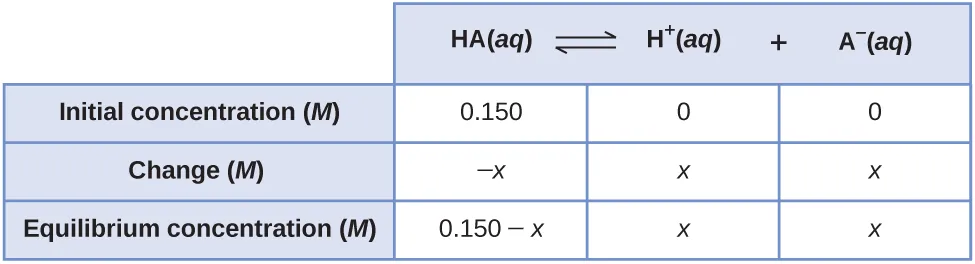Setting up and solving the quadratic equation gives

$Kc=[H+][A−][HA]=(x)(x)(0.150−x)=6.80×10−4Kc=[H+][A−][HA]=(x)(x)(0.150−x)=6.80×10−4$
13.110
$x2+6.80×10−4x−1.02×10−4=0x2+6.80×10−4x−1.02×10−4=0$
13.111
$x=−6.80×10−4±(6.80×10−4)2−(4)(1)(−1.02×10−4)(2)(1)x=−6.80×10−4±(6.80×10−4)2−(4)(1)(−1.02×10−4)(2)(1)$
13.112
$x=0.00977Mor−0.0104Mx=0.00977Mor−0.0104M$
13.113

Using the positive (physical) root, the equilibrium concentrations are

$[HA]=0.150−x=0.140M[HA]=0.150−x=0.140M$
13.114
$[H+]=[A−]=x=0.00977M[H+]=[A−]=x=0.00977M$
13.115

A less obvious way to solve the problem would be to assume all the HA ionizes first, then the system comes to equilibrium. This could be called the “all product” starting point. Assuming all of the HA ionizes gives

$[HA]=0.150−0.150=0M[HA]=0.150−0.150=0M$
13.116
$[H+]=0+0.150=0.150M[H+]=0+0.150=0.150M$
13.117
$[A−]=0+0.150=0.150M[A−]=0+0.150=0.150M$
13.118

Using these as initial concentrations and “y” to represent the concentration of HA at equilibrium, this is the ICE table for this starting point.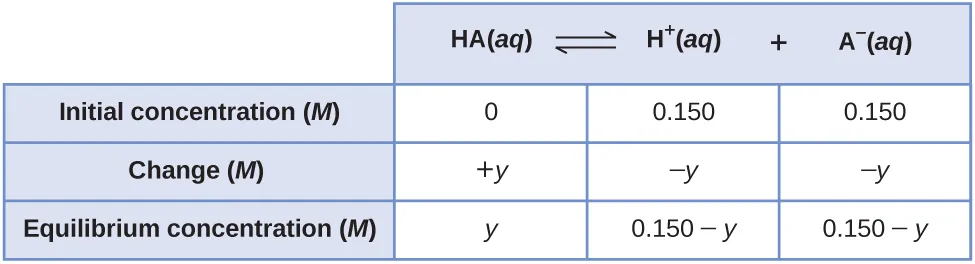Setting up and solving the quadratic equation gives

$Kc=[H+][A−][HA]=(0.150−y)(0.150−y)(y)=6.80×10−4Kc=[H+][A−][HA]=(0.150−y)(0.150−y)(y)=6.80×10−4$
13.119
$6.80×10−4y=0.0225−0.300y+y26.80×10−4y=0.0225−0.300y+y2$
13.120

Retain a few extra significant figures to minimize rounding problems.

$y2−0.30068y+0.022500=0y2−0.30068y+0.022500=0$
13.121
$y=0.30068±(0.30068)2−(4)(1)(0.022500)(2)(1)y=0.30068±(0.30068)2−(4)(1)(0.022500)(2)(1)$
13.122
$y=0.30068±0.0202102y=0.30068±0.0202102$
13.123

Rounding each solution to three significant figures gives

$y=0.160Mory=0.140My=0.160Mory=0.140M$
13.124

Using the physically significant root (0.140 M) gives the equilibrium concentrations as

$[HA]=y=0.140M[HA]=y=0.140M$
13.125
$[H+]=0.150−y=0.010M[H+]=0.150−y=0.010M$
13.126
$[A−]=0.150−y=0.010M[A−]=0.150−y=0.010M$
13.127

Thus, the two approaches give the same results (to three decimal places), and show that both starting points lead to the same equilibrium conditions. The “all reactant” starting point resulted in a relatively small change (x) because the system was close to equilibrium, while the “all product” starting point had a relatively large change (y) that was nearly the size of the initial concentrations. It can be said that a system that starts “close” to equilibrium will require only a ”small” change in conditions (x) to reach equilibrium.

Recall that a small Kc means that very little of the reactants form products and a large Kc means that most of the reactants form products. If the system can be arranged so it starts “close” to equilibrium, then if the change (x) is small compared to any initial concentrations, it can be neglected. Small is usually defined as resulting in an error of less than 5%. The following two examples demonstrate this.

### Example 13.9

#### Approximate Solution Starting Close to Equilibrium

What are the concentrations at equilibrium of a 0.15 M solution of HCN?
$HCN(aq)⇌H+(aq)+CN−(aq)Kc=4.9×10−10HCN(aq)⇌H+(aq)+CN−(aq)Kc=4.9×10−10$
13.128

#### Solution

Using “x” to represent the concentration of each product at equilibrium gives this ICE table.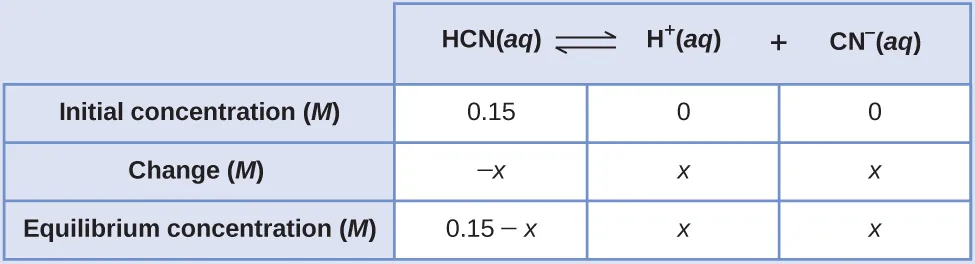The exact solution may be obtained using the quadratic formula with

$Kc=(x)(x)0.15−xKc=(x)(x)0.15−x$
13.129

solving

$x2+4.9×10−10−7.35×10−11=0x2+4.9×10−10−7.35×10−11=0$
13.130
$x=8.56×10−6M(3 sig. figs.)=8.6×10−6M(2 sig. figs.)x=8.56×10−6M(3 sig. figs.)=8.6×10−6M(2 sig. figs.)$
13.131

Thus [H+] = [CN] = x = 8.6 $××$ 10–6 M and [HCN] = 0.15 – x = 0.15 M.

In this case, chemical intuition can provide a simpler solution. From the equilibrium constant and the initial conditions, x must be small compared to 0.15 M. More formally, if $x≪0.15,x≪0.15,$ then 0.15 – x ≈ 0.15. If this assumption is true, then it simplifies obtaining x

$Kc=(x)(x)0.15−x≈x20.15Kc=(x)(x)0.15−x≈x20.15$
13.132
$4.9×10−10=x20.154.9×10−10=x20.15$
13.133
$x2=(0.15)(4.9×10−10)=7.4×10−11x2=(0.15)(4.9×10−10)=7.4×10−11$
13.134
$x=7.4×10−11=8.6×10−6Mx=7.4×10−11=8.6×10−6M$
13.135

In this example, solving the exact (quadratic) equation and using approximations gave the same result to two significant figures. While most of the time the approximation is a bit different from the exact solution, as long as the error is less than 5%, the approximate solution is considered valid. In this problem, the 5% applies to IF (0.15 – x) ≈ 0.15 M, so if

$x0.15×100%=8.6×10−60.15×100%=0.006%x0.15×100%=8.6×10−60.15×100%=0.006%$
13.136

is less than 5%, as it is in this case, the assumption is valid. The approximate solution is thus a valid solution.

What are the equilibrium concentrations in a 0.25 M NH3 solution?
$NH3(aq)+H2O(l)⇌NH4+(aq)+OH−(aq)Kc=1.8×10−5NH3(aq)+H2O(l)⇌NH4+(aq)+OH−(aq)Kc=1.8×10−5$
13.137

Assume that x is much less than 0.25 M and calculate the error in your assumption.

$[OH−]=[NH4+]=0.0021M;[OH−]=[NH4+]=0.0021M;$ [NH3] = 0.25 M, error = 0.84%

The second example requires that the original information be processed a bit, but it still can be solved using a small x approximation.

### Example 13.10

#### Approximate Solution After Shifting Starting Concentration

Copper(II) ions form a complex ion in the presence of ammonia
$Cu2+(aq)+4NH3(aq)⇌Cu(NH3)42+(aq)Kc=5.0×1013=[Cu(NH3)42+][Cu2+(aq)][NH3]4Cu2+(aq)+4NH3(aq)⇌Cu(NH3)42+(aq)Kc=5.0×1013=[Cu(NH3)42+][Cu2+(aq)][NH3]4$
13.138

If 0.010 mol Cu2+ is added to 1.00 L of a solution that is 1.00 M NH3 what are the concentrations when the system comes to equilibrium?

#### Solution

The initial concentration of copper(II) is 0.010 M. The equilibrium constant is very large so it would be better to start with as much product as possible because “all products” is much closer to equilibrium than “all reactants.” Note that Cu2+ is the limiting reactant; if all 0.010 M of it reacts to form product the concentrations would be
$[Cu2+]=0.010−0.010=0M[Cu2+]=0.010−0.010=0M$
13.139
$[Cu(NH3)42+]=0.010M[Cu(NH3)42+]=0.010M$
13.140
$[NH3]=1.00−4×0.010=0.96M[NH3]=1.00−4×0.010=0.96M$
13.141

Using these “shifted” values as initial concentrations with x as the free copper(II) ion concentration at equilibrium gives this ICE table.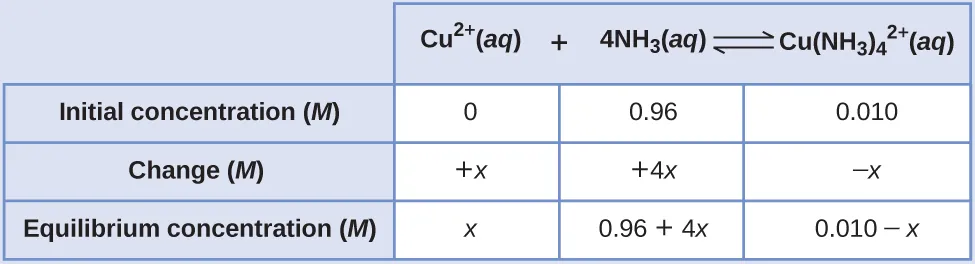Since we are starting close to equilibrium, x should be small so that

$0.96+4x≈0.96M0.96+4x≈0.96M$
13.142
$0.010−x≈0.010M0.010−x≈0.010M$
13.143
$Kc=(0.010−x)x(0.96−4x)4≈(0.010)x(0.96)4=5.0×1013Kc=(0.010−x)x(0.96−4x)4≈(0.010)x(0.96)4=5.0×1013$
13.144
$x=(0.010)Kc(0.96)4=2.4×10−16Mx=(0.010)Kc(0.96)4=2.4×10−16M$
13.145

Select the smallest concentration for the 5% rule.

$2.4×10−160.010×100%=2×10−12%2.4×10−160.010×100%=2×10−12%$
13.146

This is much less than 5%, so the assumptions are valid. The concentrations at equilibrium are

$[Cu2+]=x=2.4×10−16M[Cu2+]=x=2.4×10−16M$
13.147
$[NH3]=0.96−4x=0.96M[NH3]=0.96−4x=0.96M$
13.148
$[Cu(NH3)42+]=0.010−x=0.010M[Cu(NH3)42+]=0.010−x=0.010M$
13.149

By starting with the maximum amount of product, this system was near equilibrium and the change (x) was very small. With only a small change required to get to equilibrium, the equation for x was greatly simplified and gave a valid result well within the 5% error maximum.

What are the equilibrium concentrations when 0.25 mol Ni2+ is added to 1.00 L of 2.00 M NH3 solution?
$Ni2+(aq)+6NH3(aq)⇌Ni(NH3)62+(aq)Kc=5.5×108Ni2+(aq)+6NH3(aq)⇌Ni(NH3)62+(aq)Kc=5.5×108$
13.150

With such a large equilibrium constant, first form as much product as possible, then assume that only a small amount (x) of the product shifts left. Calculate the error in your assumption.

$[Ni(NH3)62+]=0.25M,[Ni(NH3)62+]=0.25M,$ [NH3] = 0.50 M, [Ni2+] = 2.9 $××$ 10–8 M, error = 1.2 $××$ 10–5%

Order a print copy

As an Amazon Associate we earn from qualifying purchases.